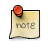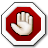Navigation C API Pages Python bindings Applications

## Matrix

Matrix is a set of helper functions on the top of linear vector to implement a resizeable 2D C array.

The number of columns and rows is not stored in the matrix and is expected to be maintaned by the user, hence most of the functions take as an argument the total number of rows and some total number of columns as well.See also matrix example usage.
Matrix allocation
`void *gp_matrix_new(size_t cols, size_t rows, size_t unit);`

Allocates a new matrix to fit `cols` * `rows` elements of size `unit`.

Initial `cols` or `rows` can be 0 as matrix can be resized later.

Returns NULL on allocation failure.

Matrix free
`void gp_matrix_free(void *self);`

Frees the matrix. Passing NULL as `self` is no-op.

Matrix index
```size_t gp_matrix_idx(size_t rows, size_t col, size_t row)

/* Example usage */
int *matrix = gp_matrix_new(10, 10, sizeof(int));

...

matrix[gp_matrix_idx(10, 5, 5)] = 42;
...```

Computes a matrix index based the total number of matrix `rows`.

Matrix cols and rows insertion
```void *gp_matrix_cols_ins(void *self, size_t rows, size_t col, size_t length)
void *gp_matrix_rows_ins(void *self, size_t cols, size_t rows, size_t row, size_t length)```

Inserts length columns/rows at given offset `col` or `row`.

The newly inserted columns/rows are set to 0.

May return NULL if underlying allocation has failed.

Returns NULL if `col` or `row` offset is out of the matrix.Returns a new pointer to the matrix!
Matrix cols and rows deletion
```void *gp_matrix_cols_del(void *self, size_t rows, size_t col, size_t length)
void *gp_matrix_rows_del(void *self, size_t cols, size_t rows, size_t row, size_t length)```

Deletes lenght columns/rows at a given offset `col` or `row`.

Returns NULL if `col` or `row` offset is out of the matrix.Returns a new pointer to the matrix!